Science, Maths & Technology

### Become an OU studentIT: device to device communication

Start this free course now. Just create an account and sign in. Enrol and complete the course for a free statement of participation or digital badge if available.

# 2.5 Working with scientific notation using the Windows calculator

Most electronic calculators will enable you to perform calculations on numbers expressed in scientific notation. This section will take you through an exercise using the Windows calculator to perform the following calculation: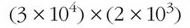Notice how we have placed the two terms in brackets. Often this is done to ensure that each step of a calculation is done in the right order. Here it isn't strictly necessary to include brackets since, when multiplying together a number of terms, the result is the same regardless of order. However, brackets do help to tidy things up and show which terms belong together.

Start the Windows calculator running on your computer (go to the Start menu and select Programs > Accessories > Calculator) then follow each step shown in the table below. (The > symbol represents the small black triangle shown on the right of a menu item, which indicates a sub-menu.)

Step Action Calculator display
1Make sure the Windows calculator is in Scientific mode by selecting Scientific from the View menu.0.
2Enter 3.3.
3Click the Exp button which you will find in the left half of the calculator keyboard. ('Exp' stands for 'exponent'.) This tells the calculator that the next number you enter will be a power of 10 and that you are working in scientific notation. The 'e' now showing on the display indicates that the number you have entered is displayed in scientific notation.3.e + 0
4Enter 4. The display is now showing the equivalent of 3×104.You have now finished entering the term in the first set of brackets.3.e + 4
5Click the multiply (*) button. This tells the calculator that you want to multiply the number showing in the display by some other number. The display now changes to show the number you have entered in its full form (3×104= 30000).30000.
6You will now start to enter the term in the second set of brackets. Enter 2.2.
7Click the Exp button.2.e+0
8Enter 3. The display is now showing the equivalent of 2×103. You have now finished entering the term in the second set of brackets.2.e+3
9Click the equal (=) button. This tells the calculator that you want it to display the result of the calculation. This is shown in its full form.60000000.
10Finally, you can force the calculator to display the result of the calculation in scientific notation. Do this by clicking the F-E button which you will find in the left half of the calculator keyboard. ('F-E' stands for 'fixed to exponent') The display is now showing the result in the 'shorthand' form which you can interpret as 6×107. 6.e+7

The result of the above exercise shows that (3×104)×(2×103)=6×107. Simple calculations like these can, in fact, be carried out quite easily without the need for a calculator, as we will explain below.

In calculations where terms are multiplied, the order of the terms isn't important and will not affect the result, so: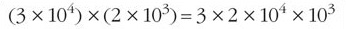Writing this in full would give: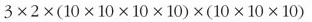Since: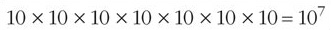we hope you can see that: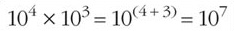and therefore: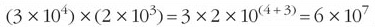So when multiplying together two or more terms expressed in scientific notation, a shortcut to the result is to add the powers.

Sometimes the calculation will require a little more manipulation in order to express the result in scientific notation. For example: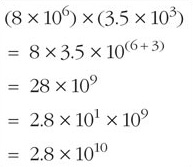Similar principles can be used when dividing terms expressed in scientific notation. A shortcut to the result is to subtract the powers.

To demonstrate we'll evaluate (3×104) divided by (2×103):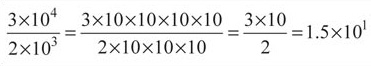We hope you can see from this that: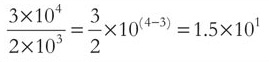## Activity 9: self-assessment

1. Use the 'short-cut' method to evaluate the following, write down your answers and then check your results using the Windows calculator.

1. (5×102)×(7×103)

2.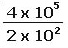2. Use the Windows calculator to evaluate the following, write down your answers correct to three significant figures.

1. (8.55×104)×(5.04×106)

2. (5.24×102)×(7.53×103)